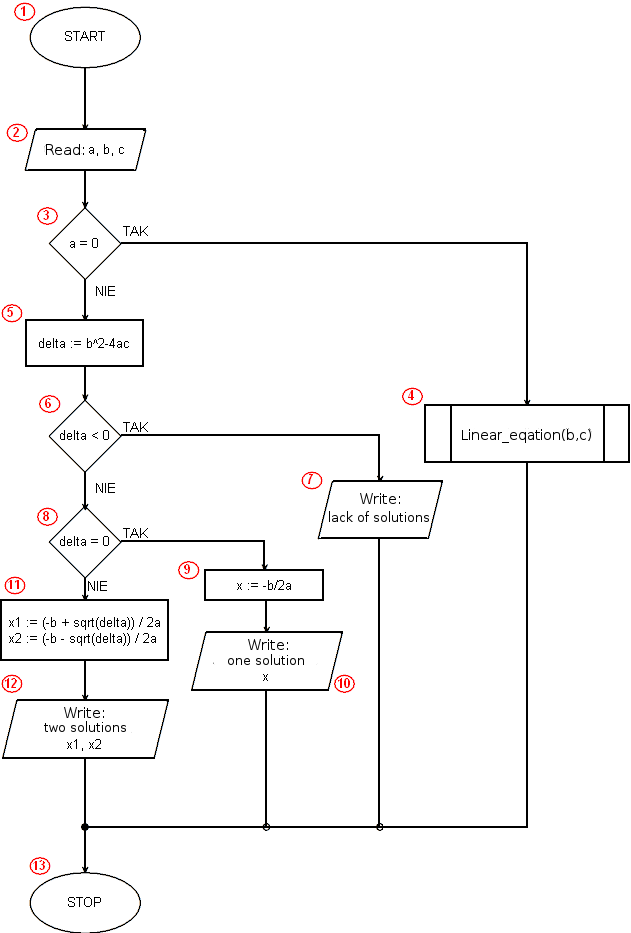# Implementation of the algorithm solving a quadratic equation in C ++

<<algorithm```#include <iostream>
#include <math.h>
using namespace std;
void linear(float a, float b){
if(a==0)
if(b==0)
cout<<"identity equation"<<endl;
else
cout<<"contrary equation"<<endl;
else{
float x;
x=-b/a;
cout<<"x = "<<x;
}
}
int main(void){
float a, b, c;
cout<<"a = ";
cin>>a;
cout<<"b = ";
cin>>b;
cout<<"c = ";
cin>>c;
if(a==0)
linear(b, c);
else{
float delta;
delta=b*b-4*a*c;
if(delta<0)
cout<<"lack of solutions"<<endl;
else
if(delta==0){
float x;
x=-b/(2*a);
cout<<"one solution x = "<<x<<endl;
} else{
float x1, x2;
x1=(-b+sqrt(delta))/(2*a);
x2=(-b-sqrt(delta))/(2*a);
cout<<"x1 = "<<x1<<endl;
cout<<"x2 = "<<x2<<endl;
}
}
}```

 Program line Description Block algorithm 1 We attach the iostream header file (input-output streams). This is necessary because in the further part of the program you will want to be able to write on the screen (cout) and load input data from the keyboard (cin). - 2 We attach the header file of the math library. In the program, we will use the sqrt function (square root) defined in this library. - 3 We inform the compiler that we will use in the program names defined in the std namespace (they will be cin, cout, endl), if we did not, it would be necessary to use a longer record of qualified names (std::cin, std::cout, std::endl). - 4 - 15 We declare and define a function named linear that implements the algorithm of solving a linear equation. 4 16 The beginning of the main function. The program execution in C ++ starts with the main function. 1 17 We declare variables a, b, c; each variable must be declared before use. - 18 - 23 On the screen, we print the prompts for the user and load the values of the coefficients a, b and c entered into the corresponding, previously declared, variables. 2 24 We check if the coefficient a is equal to 0. If this is the case with the linear equation. 3 25 In this line we will only find ourselves if a = 0, ie when the equation is a linear equation. We call the function linear(b, c) that realizes the algorithm of solving a linear equation. It is worth noting that the coefficient at the first power of the variable x is marked with the symbol b, and the free coefficient c (traditionally in the linear equation, a and b are used respectively). 4 26 else, i.e. otherwise. At this point, the solution of the equation begins, when a ≠ 0, in other words when we actually deal with a quadratic equation. - 27 - 28 We declare and calculate the value of the delta variable. 5 29 We check if delta < 0. 6 30 When delta < 0, we print an appropriate message about the lack of solutions. 7 31 Here the program block begins when the delta is not less than zero, ie when the equation has solutions (one or two). - 32 We check if delta = 0. 8 33 We declare the variable x in which we place the solution (one double solution - delta = 0). - 34 We calculate and write in the x variable the solution of the equation. 9 35 We display the calculated result. 10 36 We start the program block executed in the situation when the condition delta = 0 (and earlier delta <0 and a ≠ 0) was not met. - 37 We declare variables x1 and x2, in which we will place solutions of the equation. - 38 - 39 We calculate solutions and their values we assign to variables x1 and x2. 11 40 - 41 We display the calculated result - two solutions of the quadratic equation. 12 42 - 43 We close the blocks of compound instructions. - 44 A brace ending the main function, and thus the implementation of the entire program. 13